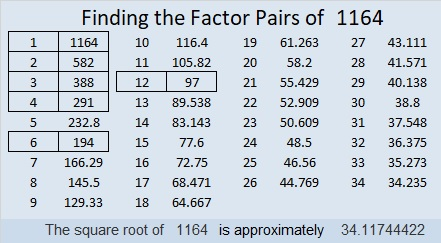# 1164 Mathematics at Corvin Castle

In the forests of Transylvania in what is now Hunedoara, Romania, a large, beautiful, well-restored, and fascinating castle awaits. At Corvin Castle, you can see how enemies were tortured and royal friends were entertained in the days of kings and knights. However, the room that intrigued me the most was full of mathematics. I didn’t take a picture of every geometric shape that graced its walls; I only took a few. You will have to go there yourself to see all the wonderful mathematical artwork. I thoroughly enjoyed myself!I knew that fellow mathematical puzzle maker, Simona Prilogan,  was from Romania, but I didn’t realize until after I returned home that this castle is in her hometown! She recently wrote about the castle and her experience growing up in Hunedoara. As a child, she was chosen to write a poem about Romania’s president and present the poem at this very castle.

Now I’ll tell you a little something about the number 1164:

• 1164 is a composite number.
• Prime factorization: 1164 = 2 × 2 × 3 × 97, which can be written 1164 = 2² × 3 × 97
• The exponents in the prime factorization are 2, 1, and 1. Adding one to each and multiplying we get (2 + 1)(1 + 1)(1 + 1) = 3 × 2 × 2 = 12. Therefore 1164 has exactly 12 factors.
• Factors of 1164: 1, 2, 3, 4, 6, 12, 97, 194, 291, 388, 582, 1164
• Factor pairs: 1164 = 1 × 1164, 2 × 582, 3 × 388, 4 × 291, 6 × 194, or 12 × 97
• Taking the factor pair with the largest square number factor, we get √1164 = (√4)(√291) = 2√291 ≈ 34.1174441164 is the sum of consecutive prime numbers FOUR different ways:
It’s the sum of the eighteen prime numbers from 29 to 103.
97 + 101 + 103 + 107 + 109 + 113 + 127 + 131+ 137 + 139 = 1164; that’s ten consecutive primes,
281 + 283 + 293 + 307 = 1164; that’s four consecutive primes, and
577 + 587 = 1164; that’s two consecutive primes.

1164 is the hypotenuse of a Pythagorean triple:
780-864-1164 which is 12 times (65-72-97)

1164 is palindrome 969 in BASE 11 because 9(11²) + 6(11) + 9(1) = 1164,
and it’s 345 in BASE 19 because 3(19²) + 4(19) + 5(1) = 1164